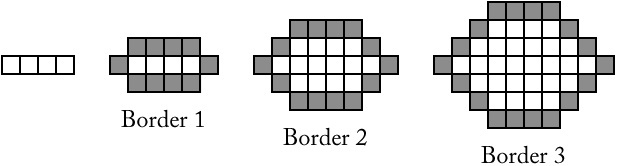# Kitchen Floor Tiles

Alignments to Content Standards: A-SSE.A.1

Fred has some colored kitchen floor tiles and wants to choose a pattern using them to make a border around white tiles. He generates patterns by starting with a row of four white tiles. He surrounds these four tiles with a border of colored tiles (Border 1). The design continues as shown below:Fred writes the expression $4(b-1)+10\,$ for the number of tiles in each border, where $b$ is the border number, $b \ge 1$.

1. Explain why Fred's expression is correct.
2. Emma wants to start with ﬁve tiles in a row. She reasons, “Fred started with four tiles and his expression was $4(b-1)+10$. So if I start with ﬁve tiles, the expression will be $5(b-1)+10$. Is Emma’s statement correct? Explain your reasoning.
3. If Emma starts with a row of $n$ tiles, what should the expression be?

## IM Commentary

The purpose of this task is to give students practice in reading, analyzing, and constructing algebraic expressions, attending to the relationship between the form of an expression and the context from which it arises. The context here is intentionally thin; the point is not to provide a practical application to kitchen floors, but to give a framework that imbues the expressions with an external meaning.

Analyzing and generalizing geometric patterns such as the one in this task may be familiar to students from work in previous grades, so part a may be a review of that process. It requires students to make use of the structure in the expression (MP7), to notice and express the regularity in the repeated geometric construction (MP8), and to explain and justify the reasoning of others (MP3). Part b requires a deeper analysis of the expression, identifying the referents for its various parts (MP7). Students may still need guidance in writing the formula for part c since it introduces a second variable.

This task includes an experimental GeoGebra worksheet, with the intent that instructors might use it to more interactively demonstrate the relevant content material. The file should be considered a draft version, and feedback on it in the comment section is highly encouraged, both in terms of suggestions for improvement and for ideas on using it effectively. The file can be run via the free online application GeoGebra, or run locally if GeoGebra has been installed on a computer.

• ## Solution

1. For Border 1 tiles are added above and below the original 4 tiles—a total of 8 tiles—and a tile is added to each end of the row of original tiles—2 additional tiles—for a total of 10 tiles.

For Border 2, we have 4 additional tiles needed to fill in the corners of the diagram (1 tile for each corner gap), plus the original 10 tiles coming from the top and bottom rows of 4 tiles each and the 2 end tiles, $$4 + 10\text{ colored tiles in Border 2.}$$ For Border 3, there are now 2 tiles in each of the 4 corners, plus the same 10 tiles from the top, bottom and ends, so there are $$4(2) + 10 \text{ colored tiles in Border 3.}$$ For Border 4, we have 3 tiles in each corner, for a total of $$4(3) +10 \text{ colored tiles in Border 4.}$$The following table illustrates the pattern:

Border number Number of tiles in the border
$1$ $10$
$2$ $4(1) + 10$
$3$ $4(2) + 10$
$4$ $4(3) + 10$
$\vdots$
$b$ $4(b - 1) + 10$

In Border $b$ there are $b-1$ extra tiles needed at each of the 4 corners, so the number of border tiles needed is given by Fred's expression $$4(b-1) + 10.$$

2. In part a, the number 10 comes from the top row of tiles, the bottom row of tiles, and the 2 tiles on the ends of the original 4 tiles. If Emma starts with 5 tiles, that number would change to 12: 5 tiles above the originals, 5 tiles below the originals, and 1 tile on each end. Emma's formula is not correct. She has incorrectly assumed that the 4 in Fred's formula came from the number of tiles in the beginning row, when it actually comes from the number of corners in the diagram itself. Regardless of the number of tiles in the beginning row, there will always be 4 corners to be filled. If Emma wants a formula for the number of tiles in each border starting with 5 tiles in the original row, she could use $$t=4(b-1)+12$$
3. In general, the number of tiles added at the top and the bottom in each border will always match the number in the original row ($n$) and there will always be 1 tile added to each end so if there are $n$ tiles in the original row, the constant in the expression will be $2n + 2$.

The number of tiles needed for each corner will remain the same regardless of the number of tiles in the original row. If $b$ is the number of the border, $4(b – 1)$ corner tiles are needed. So if Emma starts with a row of $n$ tiles, the number of tiles in the Border $b$ is $$4(b-1)+(2n+2).$$